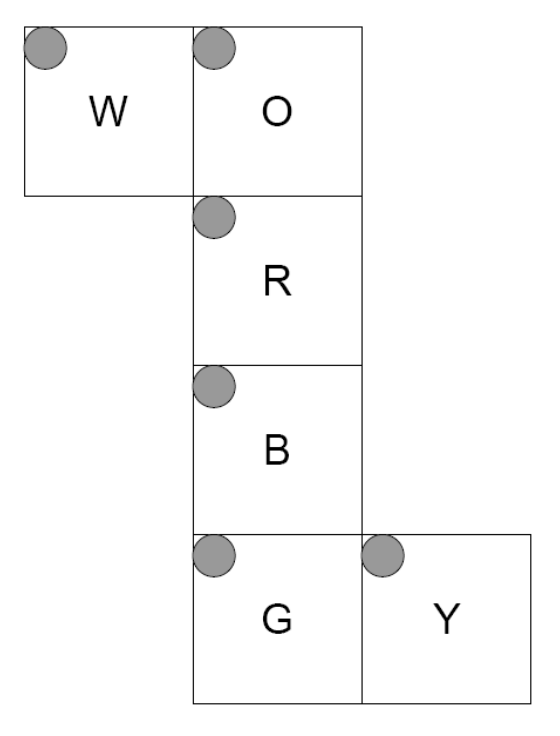시간 제한메모리 제한제출정답맞힌 사람정답 비율
1 초 128 MB111100.000%

## 문제You are a secret agent for the Eternally Indebted External Intelligence Office (EIEIO) of the country of Nomoneo. Headquarters has disguised your one-time pad for encrypted communications in the form of a Rubik's Cube®. (For those of you unfamiliar with the puzzle, a Rubik's Cube® comes in the form of a cube where each face is divided into three rows and three columns (nine “squares”). Any of the six faces of the cube may be rotated either clockwise or counterclockwise, which also rotates the three nearest squares on each adjoining face onto a new face, respectively. When solved (or taken from the factory packaging), each face of the cube contains squares of only one color. There is no way to change the relationship between the colors of the central squares on each face.) The cube has been pre-scrambled and you are to apply a certain set of moves to the cube based on the message you want to return.

This diagram provides the relationship between the sides of the cube as well as the orientation of the faces for the purposes of input and output. It should be viewed as an unfolded cube with the text on the outside. The faces are indicated by the color of the central subdivision (square) and are White, Orange, Red, Blue, Green, and Yellow. The corner with the dot is the top left corner for purposes of input and output.

## 입력

Your program should read the input data from the file, which consists of several cases. The first line of the input will give the number of these input cases (as a decimal integer without any punctuation), which will be greater than or equal to 1 and less than or equal to 10,000. Each case consists of three lines giving the initial state of the puzzle cube and one line giving the rotations you must apply to reach the appropriate final state of the cube.

Each of the three lines giving the initial state of the cube consists of 18 letters with a single space between each pair of letters. There is no space between the last letter and the end of the line. Each of the letters is 'W', 'O', 'R', 'B', 'G', or 'Y' indicating the respective color. The faces are listed from left to right in the order (by central square color) White, Orange, Red, Blue, Green, Yellow. Thus, the first three columns of letters give the state of the “White” face, and so forth.

The fourth line of the case gives the manipulations that you must apply to the cube. Each manipulation consists of a single letter as above ('W', 'O', 'R', 'B', 'G', or 'Y') indicating which face (selected by the color of the center) you should rotate. Each rotation thus indicated is a 90° clockwise rotation of the face with respect to the rest of the cube, looking at the face to be rotated. At least one and no more than 1,000 manipulations will be specified.

## 출력

Print to standard output the state of the cube after the indicated manipulations. Use the same format as for input: three lines, each containing 18 color letters separated by a single space character. Do not put a space after the final letter on the line. Print out the faces in the same order, left to right, as for input: White, Orange, Red, Blue, Green, Yellow. After each output case, print a line containing 35 '=' characters.

## 예제 입력 1

2
W W W O O O R R R B B B G G G Y Y Y
W W W O O O R R R B B B G G G Y Y Y
W W W O O O R R R B B B G G G Y Y Y
RG
Y G G Y G W Y W B R W O R W G B G G
O W R Y O B G R O R B O Y G R B Y Y
O W O G O W Y B W B R W B B O R Y R
GROWBOBGROW


## 예제 출력 1

O O O Y Y Y R R R Y Y Y G G G B Y O
W W W O O O R R R B B B G G G B Y O
B B B W W W R R R W W W G G G B Y O
===================================
W G R Y B G B R G B O W O R B R W R
G W B G O R G R R Y B B O G O Y Y B
G W R Y Y Y W W O G O W O Y B Y W O
===================================